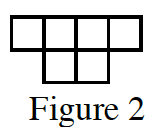Home > CC3 > Chapter 6 > Lesson 6.2.4 > Problem6-82

6-82.

Figure 2 of a tile pattern is shown at right. If the pattern grows linearly and if Figure 6 has $18$ tiles, then find a rule for the pattern. Homework Help ✎Make an $x→y$ table with the $x$-values representing the figure number and the $y$-values representing the number of tiles.

Fill in values for $x$ and $y$. What is the growth?

Work backwards. What is the starting point or the number of tiles in Figure 0?

Write an equation in $y=mx+b$ form, using the growth and starting point.

$y=3x$

Does this rule work for your table?